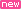# LRS 2020 Charges Calculation | LRS Calculator Know Your LRS Charges in 5 Minutes

LRS 2020 Charges Calculation  | LRS Calculator Know Your LRS Charges in 5 Minutes: Telangana state government launched the LRS scheme in 2015 to regularize the unapproved layouts. Again in 2020, the government of Telangana again issued a GO making new amendments to the earlier scheme. The major change is in the rates to be paid for regularization. Let us see how to calculate LRS fee individual plots and layouts as per the amendments made. Basic regulation charges, percentage of basic regularization charges, pro-rata open space charges etc have to be calculated first.

## LRS 2020 Charges Estimation Fee Calculation Process

1. To calculate the basic regularization fees for plots and layouts here is the fee structure decided by the Government of Telangana. If the plot is less than 100 meters, the fee is Rs.200 per square meter. For below 300 sq. meters plot, it is Rs. 400 per square meter and for below 500 sq. meter plot, it is Rs. 600 per sq. meter. If the plot is more than 500 sq.meters, per sq. meter regularization fee is Rs. 750. To calculate this fee, convert plot area into the square meter and multiply with applicable sq. meter charge.
2. Next is the calculation of LRS percentage of basic regularization charges. The charges are as follows- for up to 3000 square yards, regularization fee is 20% of the market value of the land, for below 5000 square yards it is 30% and for below 10000 square yards it is 40% of land market value and so on.
3. Now let us see how to calculate the pro-rat open space charges. For plots, if 10% open space is not available in unapproved layout, the rate is 14% of the plot value. For layouts also, 14% of the plot value is collected as pro-rate open space charges if 10% open space is not available.

Now to calculate the LRS grand total, add the basic regularization charges, percentage of basic regularization charges and also the open space charges. The total we get is the LRS fee to be paid for regularization of unapproved layouts and plots in Telangana. To regularize the plots in slum areas, irrespective of the area of the plot, the fee is Rs. 5 per square meter.

SHARE IT

 PMAYSLRS 2020PMKSNY PMEGP PMSBYS APY SSY PMJJBY PMVVY PMMY PMUY BHIM APP